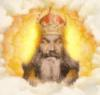# Fibonacci Numbers

No replies to this topic

### #1Guru Dudette

Guru Dudette

Prince of Dorkness

•• 835 posts

Posted 31 January 2007 - 11:09 AM

Fibonacci numbers form a sequence defined by the following formula:That is, after two starting values,each number is the sum of the two preceding numbers. The first Fibonacci numbers also denoted as Fn, for n = 0, 1,  , are:

0, 1, 1, 2, 3, 5, 8, 13, 21, 34, 55, 89, 144, 233, 377, 610, 987, 1597, 2584, 4181, 6765, 10946, 17711, 28657, 46368, 75025

Sometimes this sequence is considered to start at F1 = 1, but it is more common to include F0 = 0.

The Fibonacci numbers are named after Leonardo of Pisa, known as Fibonacci.
"I'd rather be vaguely right than precisely wrong." J.M.Keynes